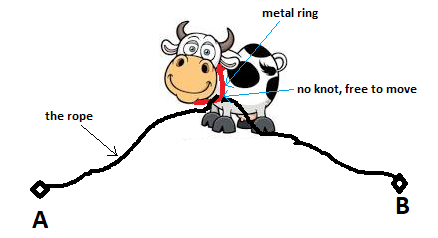# Alien InvasionArcturusAUST CSE 44th Batch Pract...
Limits 1s, 512 MB

Fisaow is a farmer. He has a large field covered with grass and wants to create some pattern or design in that field by removing grasses at certain places so that it looks like a crop circle from the sky. But he is not familiar with geometric shapes. One day he noticed that when a cow eats grass in the fields, it creates different geometric shapes based on how they are tied in the field.

Now he has $N$ cows and $N$ ropes. For every cow he will use 2 points $A_i$ and $B_i$ to tie it up. That means, one end of the rope will be tied to point $A_i$ and the other end to $B_i$. Every cow has a metal ring around its neck and the rope will go through the metal ring such a way that the cow can move freely (see the picture: there is no knot with the ring). The cows will eat grass from wherever it can go. The amount of grass each cow can eat is the area that each cow can cover.Now Fisaow has given you the points $A_i$ $(X_1, Y_1)$ and $B_i$ $(X_2, Y_2)$ the length of the ropes $L_i$ for each of the $N$ cows. Help him to find the maximum amount of grass each cow can eat.

Note: $\text{Distance}(A_i, B_i) ≤ L_i$.

## Input

Input starts with an integer $N$ ($0 < N ≤ 100000$), the number of cows. The next $N$ lines will have 5 space separated integers $X_1$, $Y_1$, $X_2$, $Y_2$, ($-10^6 ≤ X_1, Y_1, X_2, Y_2 ≤ 10^6$), coordinates of the two points, and $L$ ($0 \le L ≤ 10^7$), the length the each rope.

## Output

For each cow, print the maximum amount of grass it can eat in separate lines. Absolute errors less than $10^{-6}$ will be ignored.

## Sample

InputOutput
4
0 0 6 0 10
-4 3 8 3 20
-5 6 5 6 26
-3 -6 -3 -18 20

62.8318530718
251.3274122872
490.0884539600
251.3274122872GeometryuDebug

### Submit